# CHAPTER NEW CHAPTER Waves the BIG idea Waves

• Slides: 12CHAPTER NEW CHAPTER Waves the BIG idea Waves transfer energy and interact in predictable ways. 15. 1 Waves transfer energy. 15. 2 Waves have measurable properties. 15. 3 Waves behave in predictable ways. CHAPTER OUTLINE CHAPTER RESOURCES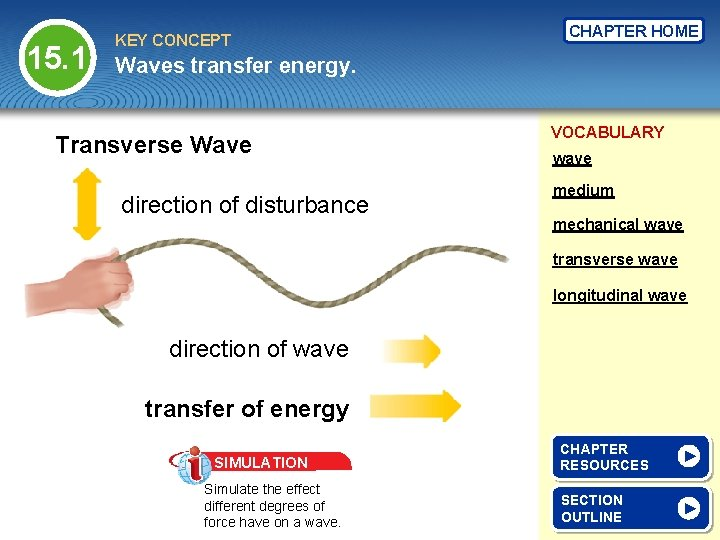15. 1 KEY CONCEPT CHAPTER HOME Waves transfer energy. Transverse Wave direction of disturbance VOCABULARY wave medium mechanical wave transverse wave longitudinal wave direction of wave transfer of energy SIMULATION Simulate the effect different degrees of force have on a wave. CHAPTER RESOURCES SECTION OUTLINE15. 1 KEY CONCEPT CHAPTER HOME Waves transfer energy. VOCABULARY wave Longitudinal Wave direction of disturbance medium mechanical wave transverse wave longitudinal wave direction of wave transfer of energy CHAPTER RESOURCES SECTION OUTLINE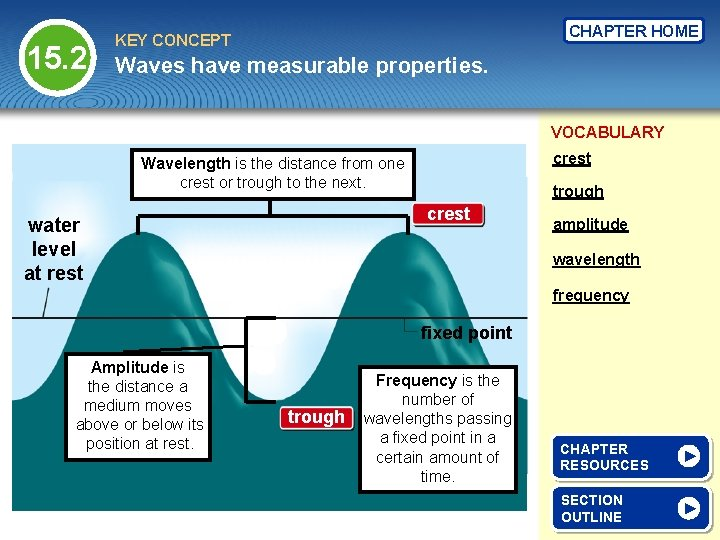15. 2 CHAPTER HOME KEY CONCEPT Waves have measurable properties. VOCABULARY crest Wavelength is the distance from one crest or trough to the next. trough crest water level at rest amplitude wavelength frequency fixed point Amplitude is the distance a medium moves above or below its position at rest. trough Frequency is the number of wavelengths passing a fixed point in a certain amount of time. CHAPTER RESOURCES SECTION OUTLINE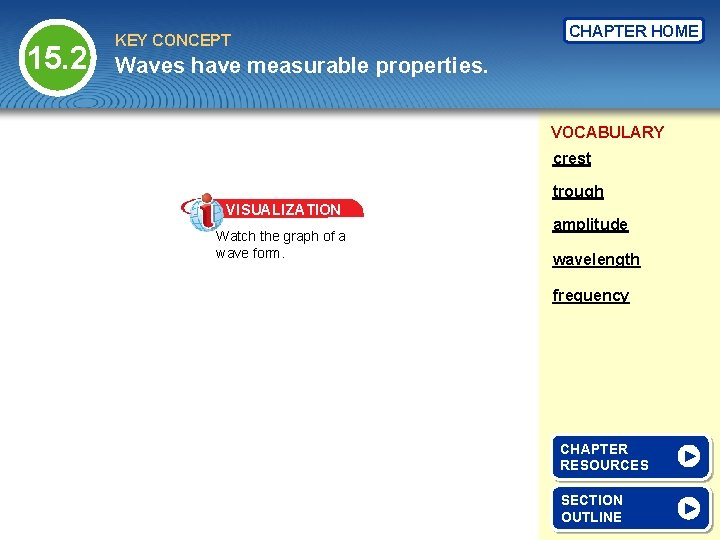15. 2 KEY CONCEPT CHAPTER HOME Waves have measurable properties. VOCABULARY crest trough VISUALIZATION Watch the graph of a wave form. amplitude wavelength frequency CHAPTER RESOURCES SECTION OUTLINE15. 3 KEY CONCEPT CHAPTER HOME Waves behave in predictable ways. VOCABULARY Reflection refraction diffraction interference CHAPTER RESOURCES SECTION OUTLINE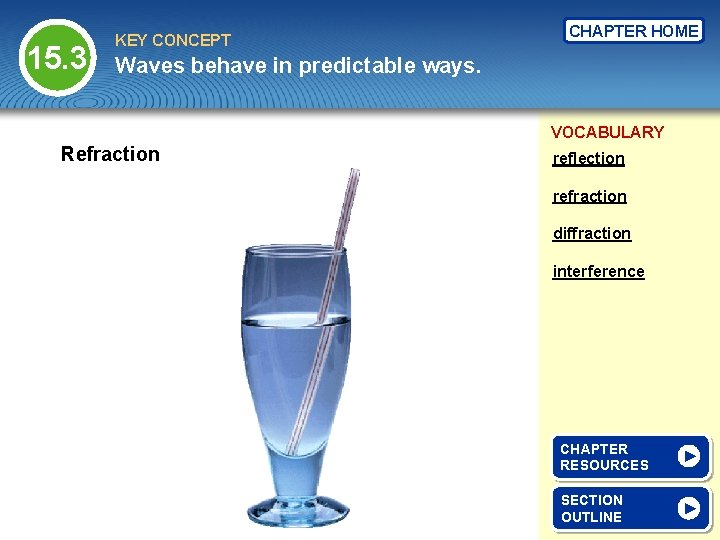15. 3 KEY CONCEPT CHAPTER HOME Waves behave in predictable ways. VOCABULARY Refraction reflection refraction diffraction interference CHAPTER RESOURCES SECTION OUTLINE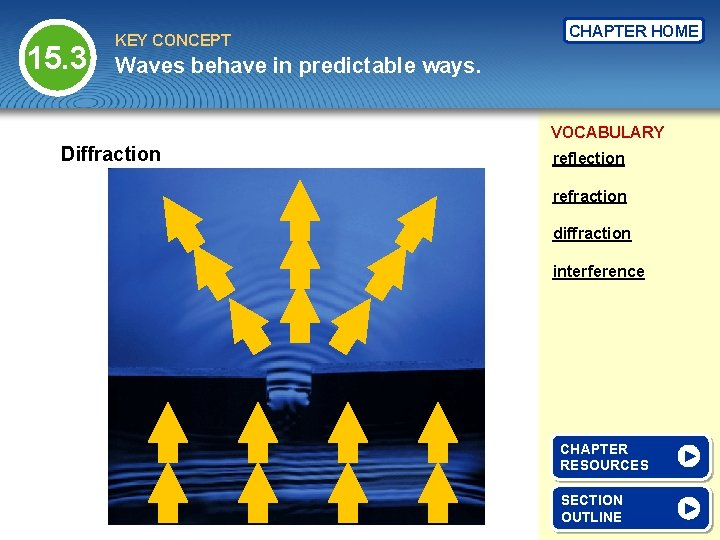15. 3 KEY CONCEPT CHAPTER HOME Waves behave in predictable ways. VOCABULARY Diffraction reflection refraction diffraction interference CHAPTER RESOURCES SECTION OUTLINE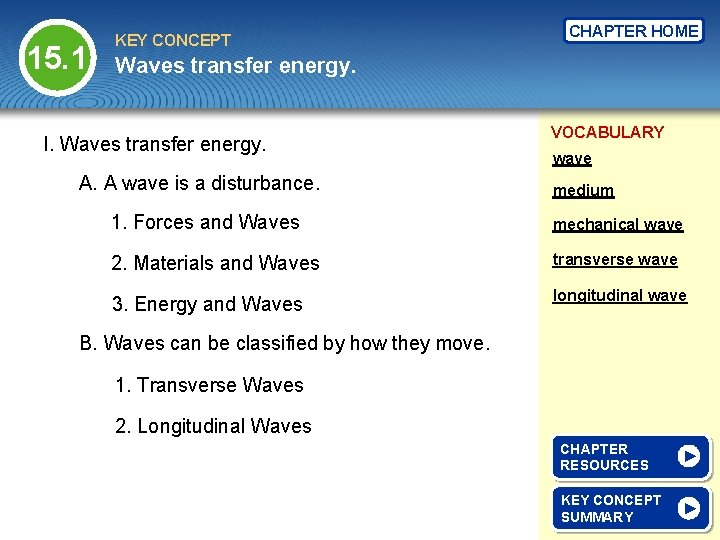15. 1 KEY CONCEPT CHAPTER HOME Waves transfer energy. I. Waves transfer energy. A. A wave is a disturbance. VOCABULARY wave medium 1. Forces and Waves mechanical wave 2. Materials and Waves transverse wave 3. Energy and Waves longitudinal wave B. Waves can be classified by how they move. 1. Transverse Waves 2. Longitudinal Waves CHAPTER RESOURCES KEY CONCEPT SUMMARY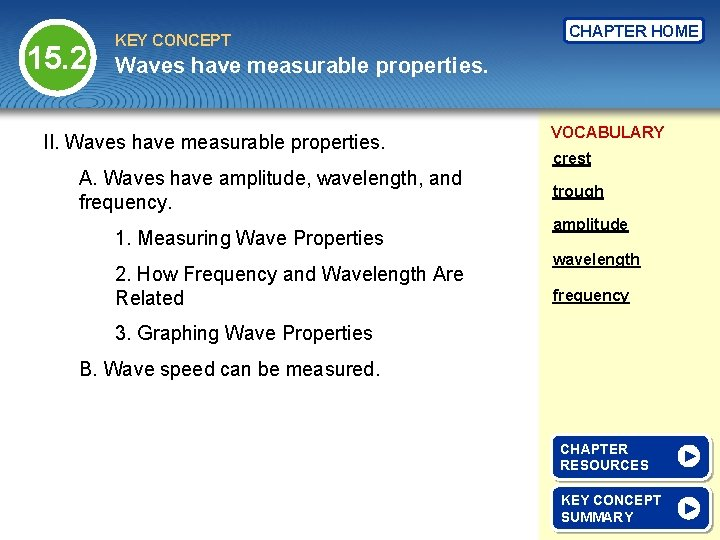15. 2 KEY CONCEPT CHAPTER HOME Waves have measurable properties. II. Waves have measurable properties. A. Waves have amplitude, wavelength, and frequency. 1. Measuring Wave Properties 2. How Frequency and Wavelength Are Related VOCABULARY crest trough amplitude wavelength frequency 3. Graphing Wave Properties B. Wave speed can be measured. CHAPTER RESOURCES KEY CONCEPT SUMMARY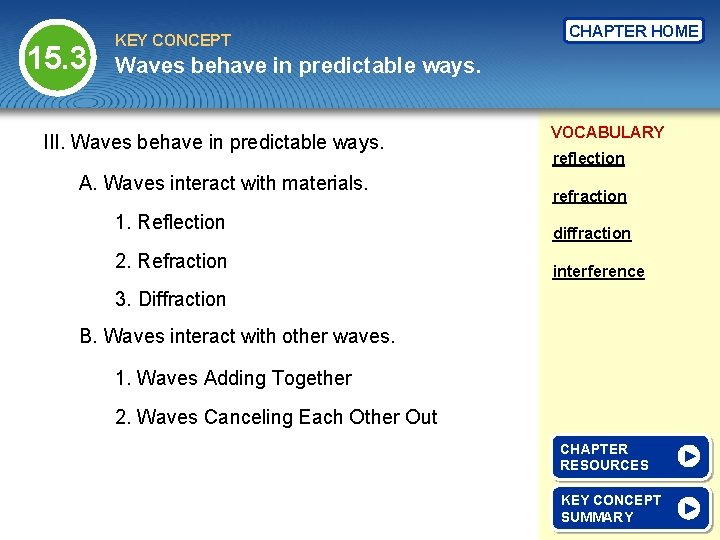15. 3 KEY CONCEPT CHAPTER HOME Waves behave in predictable ways. III. Waves behave in predictable ways. A. Waves interact with materials. 1. Reflection 2. Refraction VOCABULARY reflection refraction diffraction interference 3. Diffraction B. Waves interact with other waves. 1. Waves Adding Together 2. Waves Canceling Each Other Out CHAPTER RESOURCES KEY CONCEPT SUMMARY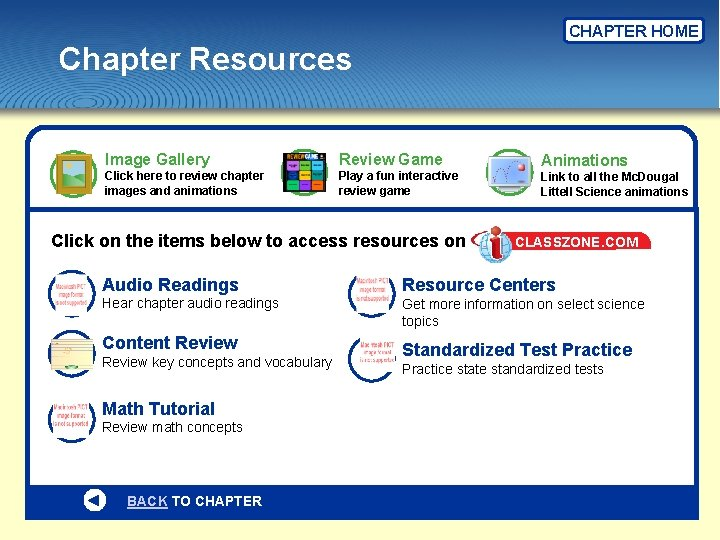CHAPTER HOME Chapter Resources Image Gallery Review Game Click here to review chapter images and animations Animations Play a fun interactive review game Link to all the Mc. Dougal Littell Science animations Click on the items below to access resources on CLASSZONE. COM Audio Readings Resource Centers Hear chapter audio readings Get more information on select science topics Content Review Standardized Test Practice Review key concepts and vocabulary Math Tutorial Review math concepts BACK TO CHAPTER Practice state standardized tests